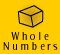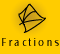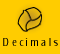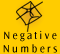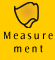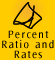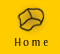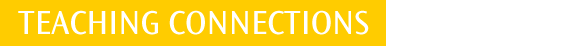| Percent | Models for percent |
| Ratio | Rates | Activities |

Teaching percent, rates and ratio

The percent, ratio and rates topic covered on the CD-ROM is fairly comprehensive and provides a general sequential approach which could be used to teach percent, ratio and rates mainly in the secondary classroom.

Children are introduced to the common language usage of percent in the early to middle years of primary. Around Years 3 to 4 they understand 100% as being a 'full thing' and 50% as a 'half. At this stage children may also be familiar with ratio terms such as '50 : 50' and understand that this conveys the idea of balance. They may also have meaning for 10 to 1, 100 to 1 and 2 to 1. Children would also have some knowledge of commonly used rates, such as 'kilometres per hour' - 'how fast are we going?' and 'cents per litre' - 'how much does petrol cost?', although they would not necessarily have an understanding how they are made up of two separate measurements.

Percent

In later primary school the precise notion of percent is developed. Children learn the simple relationship between some fractions and their percent equivalents, such as 1/2, 1/3, 1/4, 1/5, 1/8, 1/10.

At this stage children should be able to find simple percentages where the percent acts as an operator, such as 25% of .., 10% of .., 5% of .., 75% of .. 250% of ..

 For example, 75% of 60 apples = 75/100 x 60 apples = 45 apples or, 75% is 3/4 of the apples. One quarter is 15, so three quarters is 45.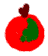They may break up the percents into more manageable pieces,

75% of 60 apples = 50% of 60 apples + 25% of 60 apples = 1/2 x 60 apples + 1/4 of 60 apples.

Another example, 250% of the current airfare is 2 1/2 times the current airfare.

Before calculators, percent problems were generally worked out using fractions. Today, however, they can also be readily worked out using decimals. The method you use depends on the numbers involved and personal preference.

For example, 75% of 60 apples = 0.75 x 60 apples = 45 apples

It is important that children see the link between the percent representation, the fraction representation and the decimal representation. By early secondary school, children should be able to work with all percents in decimal or fraction form.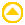Models for percent

Elastic tape measure model

We have included an example of the elastic tape measure model on this CD-ROM. This is a good linear model of percent which teachers can easily make and which can be adapted for a number of examples. For more information about this model, see Meaning and Models.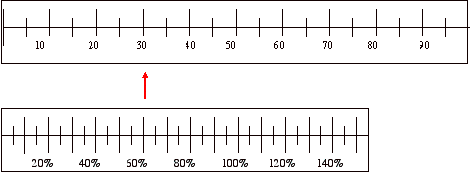Dual-scale number line

We have used the dual-scale number line as an aid to organising information when solving percent problems throughout the topic on the CD-ROM. By showing the number scale and percent scale on the one number line and representing the information we are given and the information we need to find out, we can write a proportion equation directly from the number line. See the CD-ROM content for more description and movies on how we use this model.

 Example: 30 out of 50 apples in a box are too bruised to sell. What percent of apples cannot be sold? Thinking The left side of the number line below has a percent scale. The right side of the number line has a number scale. We can label each scale using the information we are given in the problem.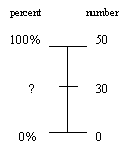We know that there are 50 apples in total, ie. 50 apples equals 100% of the apples. We know that 30 out of the 50 apples are bruised and we need to find what percent this is. In more complicated problems this dual-scale number line is a good way of organising the information we are given and to work out what information we need to find. Once we have represented the problem in this way we can write a proportion equation directly from the number line. 30/50 = ?/100 By equivalent fractions we know that 30/50 = 60/100 Therefore 60% of apples are too bruised to sell.Ratio

In late primary school children should be introduced to ratio as a part : part relationship with activities such as making up cordial.

For example, one part cordial mixed with 4 parts of water makes 5 parts of normal strength drink.

Children need to appreciate that the size of the part chosen does not affect the strength of the drink.

Ratio is a major topic in secondary school. Difficulties arise when the numbers are not simple whole numbers. The difficulties are not only computational, but conceptual.Rates

We use rates when we are measuring one quantity or amount in relation to another quantity or amount. Children will meet many rates in everyday life but they need to learn how the rate is related to the measurable quantities.

For example, children will have a feeling of speed but need to learn how it relates the distance covered to a given time.

The calculation of rates again relies on thorough understanding of multiplication and division and the use of decimals and fractions in calculation.

Percent, ratio and rates is conceptually a very difficult area, and given that it is a significant component of the secondary school curriculum up to Year 10, care must be taken to help children to consolidate their understanding. In many applications of the topic there are potentially many right and wrong ways to get the answer. Teachers need to be aware of the fact that there are a range of methods to solve most problems and need to have the flexibility to adapt these methods to suit each child's needs.

 Problems in this topic tend to have a relatively long sequence of steps and rely on an understanding of fractions (not whole numbers). Operations need to be performed on numbers between 0 and 1 which leaves plenty of room for confusion and error.Activities

 In early secondary school percent, ratio and rates make up about one third of the curriculum and there are many applications of the topics throughout secondary school.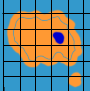Making a pie chart Scale drawing Value for money Discounts Finding the gradient of a line Drawing linear graphs through the origin Recipes and mixtures Chemical formulas and equations